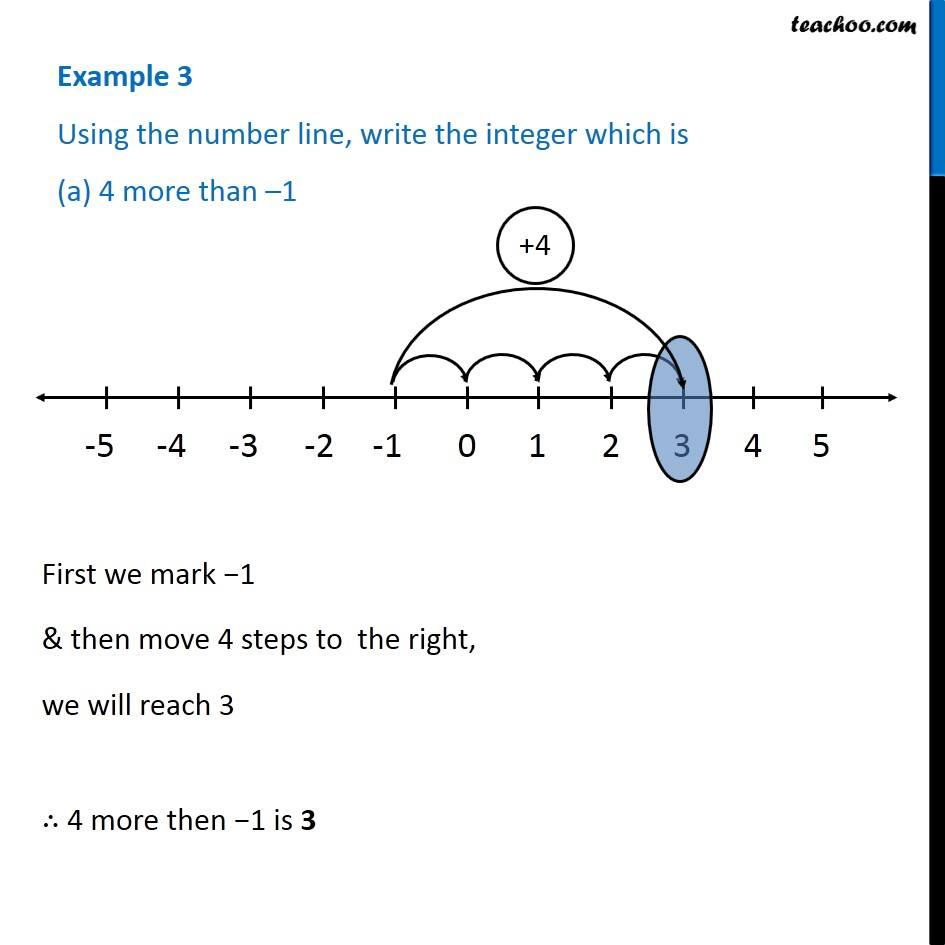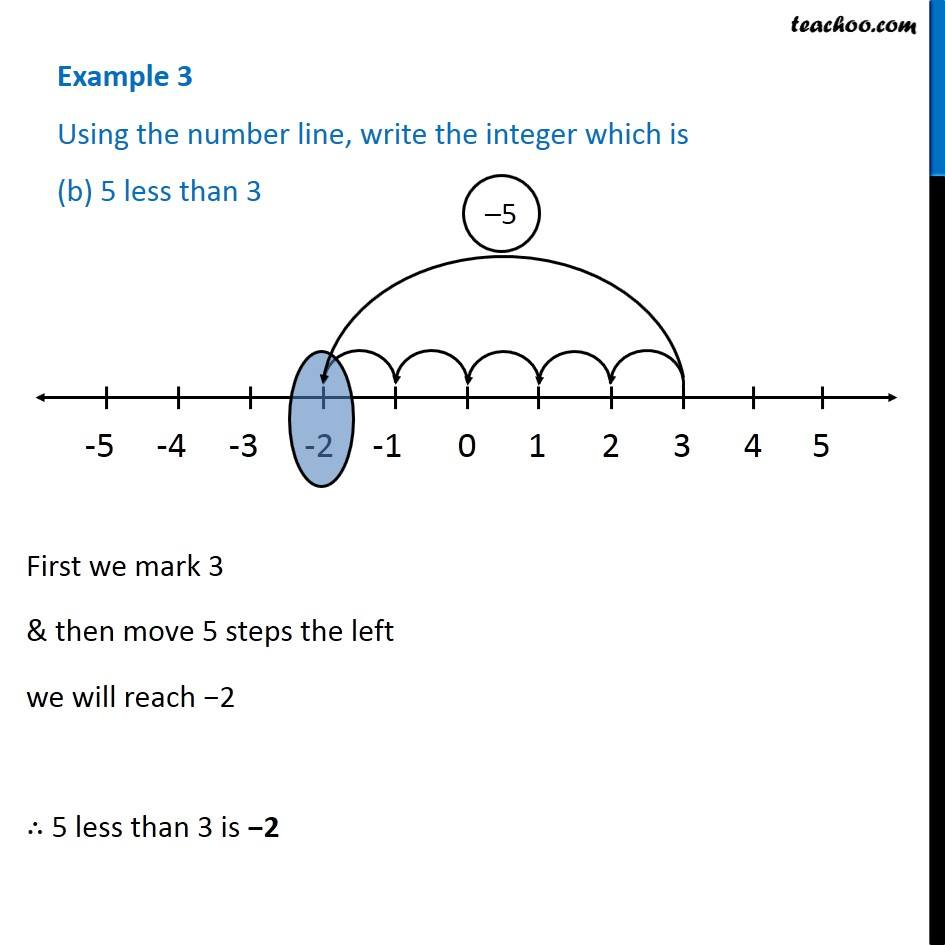1. Chapter 6 Class 6 Integers
2. Concept wise
3. Addition of integers using number line

Transcript

Example 3 Using the number line, write the integer which is (a) 4 more than –1 First we mark −1 & then move 4 steps to the right, we will reach 3 ∴ 4 more then −1 is 3 Example 3 Using the number line, write the integer which is (b) 5 less than 3 First we mark 3 & then move 5 steps the left we will reach −2 ∴ 5 less than 3 is −2

Addition of integers using number line